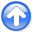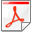Basic operators and adjointsNext: Programming linear operators Up: Reproducible Documents

Jon Claerbout

A great many of the calculations we do in science and engineering are really matrix multiplication in disguise. The first goal of this chapter is to unmask the disguise by showing many examples. Second, we see how the adjoint operator (matrix transpose) back projects information from data to the underlying model.

Geophysical modeling calculations generally use linear operators that predict data from models. Our usual task is to find the inverse of these calculations; i.e., to find models (or make images) from the data. Logically, the adjoint is the first step and a part of all subsequent steps in this inversion process. Surprisingly, in practice, the adjoint sometimes does a better job than the inverse! Better because the adjoint operator tolerates imperfections in the data and does not demand the data provide full information.

Using the methods of this chapter, you find that once you grasp the relationship between operators in general and their adjoints, you can obtain the adjoint just as soon as you have learned how to code the modeling operator.

If you will permit me a poet's license with words, I will offer you the following table of operators and their adjoints:

 matrix multiply conjugate-transpose matrix multiply convolve crosscorrelate truncate zero pad replicate, scatter, spray sum or stack spray into neighborhoods sum within bins derivative (slope) negative derivative causal integration anticausal integration add functions do integrals assignment statements added terms plane-wave superposition slant stack / beam form superpose curves sum along a curve stretch squeeze scalar field gradient negative of vector field divergence upward continue downward continue diffraction modeling imaging by migration hyperbola modeling stacking for image or velocity chop image into overlapping patches merge the patches ray tracing tomography

The left column is often called modeling,'' and the adjoint operators in the right column are often used in data processing.''

When the adjoint operator is not an adequate approximation to the inverse, then you apply the techniques of fitting and optimization explained in Chapter. These techniques require iterative use of the modeling operator and its adjoint.

The adjoint operator is sometimes called the back projection'' operator because information propagated in one direction (Earth to data) is projected backward (data to Earth model). Using complex-valued operators, the transpose and complex conjugate go together; and in Fourier analysis, taking the complex conjugate ofreverses the sense of time. With more poetic license, I say that adjoint operators undo the time and therefore, phase shifts of modeling operators. The inverse operator does also, but it also divides out the color. For example, when linear interpolation is done, then high frequencies are smoothed out; inverse interpolation must restore them. You can imagine the possibilities for noise amplification which is why adjoints are safer than inverses. But, nature determines in each application what is the best operator to use and whether to stop after the adjoint, to go the whole way to the inverse, or to stop partway.

The operators and adjoints previously shown transform vectors to other vectors. They also transform data planes to model planes, volumes, etc. A mathematical operator transforms an abstract vector'' that might be packed full of volumes of information like television signals (time series) can pack together a movie, a sequence of frames. We can always think of the operator as being a matrix, but the matrix can be truly huge (and nearly empty). When the vectors transformed by the matrices are large like geophysical data set sizes, then the matrix sizes are large squared,'' far too big for computers. Thus, although we can always think of an operator as a matrix; in practice, we handle an operator differently. Each practical application requires the practitioner to prepare two computer programs. One performs the matrix multiply, while the other multiplies by the transpose(without ever having the matrix itself in memory). It is always easy to transpose a matrix. It is less easy to take a computer program that doesand convert it to another to do, which is what we'll be doing here. In this chapter are many examples of increasing complexity. At the end of the chapter, we see a test for any program pair to see whether the operatorsandare mutually adjoint as they should be. Doing the job correctly (coding adjoints without making approximations) rewards us later when we tackle model and image-estimation applications.

Mathematicians often denote the transpose of a matrixby. In physics and engineering, we often encounter complex numbers. There, the adjoint is the complex-conjugate transposed matrix denoted. What this book calls the adjoint is more properly called the Hilbert adjoint.Basic operators and adjointsNext: Programming linear operators Up: Reproducible Documents

2014-09-27